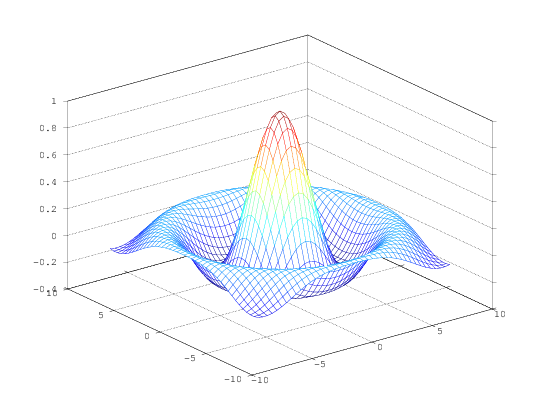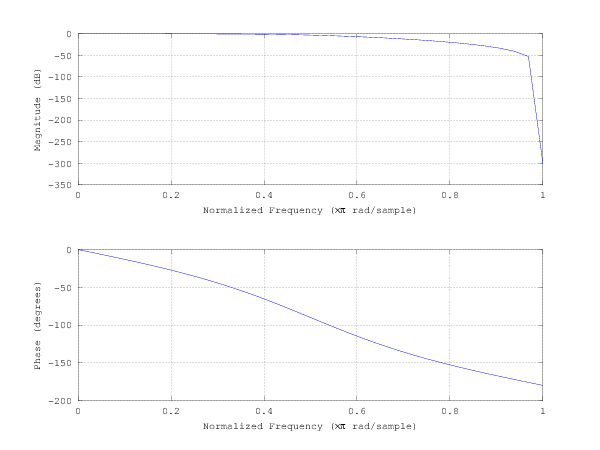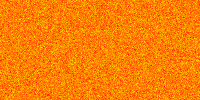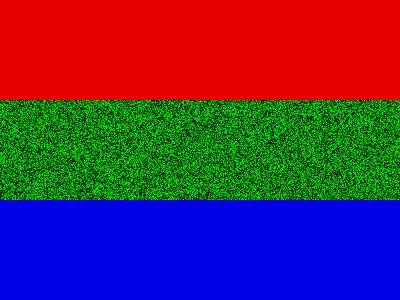# Jupyter Octave Kernel¶

Interact with Octave in Notebook. All commands are interpreted by Octave. Since this is a MetaKernel, a standard set of magics are available. Help on commands is available using the %help magic or using ? with a command.

In :
t = linspace(0,6*pi,100);
plot(sin(t))
grid on
hold on
plot(cos(t), 'r')

In :
%plot --format png
tx = ty = linspace (-8, 8, 41)';
[xx, yy] = meshgrid (tx, ty);
r = sqrt (xx .^ 2 + yy .^ 2) + eps;
tz = sin (r) ./ r;
mesh (tx, ty, tz);In :
a = [1,2,3]

a =

1   2   3


In :
b = a + 3;

In :
disp(b)

   4   5   6


The width or the height can be specified to constrain the image while maintaining the original aspect ratio.

In :
%plot -f png -w 600

% butterworth filter, order 2, cutoff pi/2 radians
b = [0.292893218813452  0.585786437626905  0.292893218813452];
a = [1  0  0.171572875253810];
freqz(b, a, 32);Multiple figures can be drawn. Note that when using imshow the image will be created as a PNG with the raw image dimensions unless the image has a title or labels.

In :
% small image
imshow(randn(100,100))

% let's change the data range
figure
imshow(randn(100,100), [-10 10])

% let's try automatic range and different size
figure
imshow(randn(100,200), [])
colormap('autumn')In :
% Create an RGB image with no title or labels
close all hidden
image = zeros(300,400,3);
image(1:100,:,1) = 0.9;  % Red
image(101:200,:,2) = rand(100,400);  % Green
image(201:300,:,3) = 0.9;  % Blue
imshow(image)In :
% image that is too small to display raw (<100 pixels)
imshow(rand(50,50))


In :
% let's add a title - this produces full figure
imshow(rand(100,100))
title('Hello')

% let's create an image next to a plot
figure
subplot(121)
plot([1,2,3])
subplot(122)
imshow(randn(100,100))


In :
%lsmagic

Available line magics:
%activity  %cd  %connect_info  %conversation  %dot  %download  %edit  %get  %help  %html  %include  %install  %install_magic  %javascript  %jigsaw  %kernel  %kx  %latex  %load  %ls  %lsmagic  %macro  %magic  %matplotlib  %parallel  %plot  %pmap  %px  %python  %reload_magics  %restart  %run  %scheme  %set  %shell

Available cell magics:
%%activity  %%brain  %%conversation  %%debug  %%dot  %%file  %%help  %%html  %%javascript  %%kx  %%latex  %%macro  %%pipe  %%processing  %%px  %%python  %%scheme  %%shell  %%show  %%time  %%tutor

In [ ]: